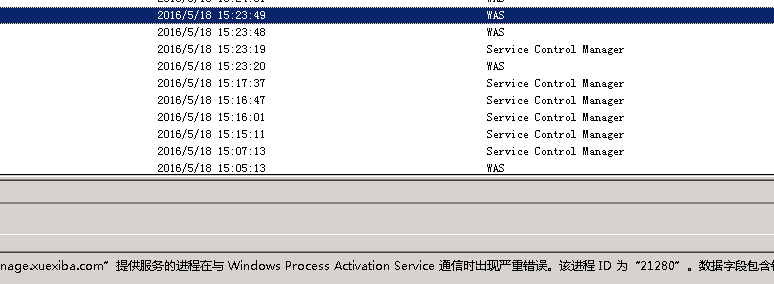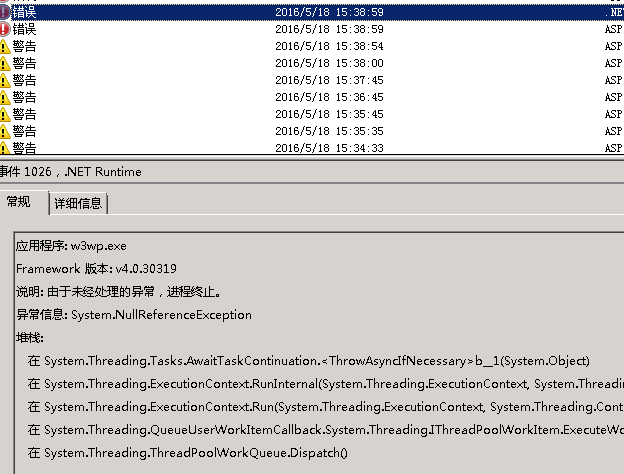#0
0
11. 云栖社区>
2. 博客>
3. 正文

mcy247 2017-12-07 23:31:00 浏览1214```   /// <summary>
/// 线程管理
/// </summary>
{
/// <summary>
/// 将在线程池上运行的指定工作排队
/// </summary>
/// <param name="action"></param>
public static void Run(Action action)
{
{
try
{
action();
}
catch (Exception ex)
{
Lind.DDD.Logger.LoggerFactory.Instance.Logger_Error(ex);
}
});

}

}```

```　　　　　　ThreadPool.QueueUserWorkItem((o) =>//w3wp会有挂机问题
{
var b = 0;
var c = 1 / b;
});

{
var b = 0;
var c = 1 / b;
});

{
var b = 0;
var c = 1 / b;

}).Start();

{
var b = 0;
var c = 1 / b;
});```